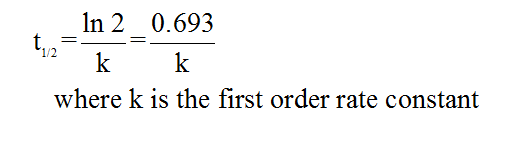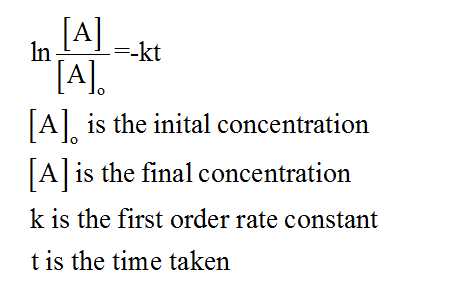# In the nuclear industry, workers use a rule of thumb that the radioactivity from any sample will be relatively harmless after 10 half-lives. Calculate the fraction of a radioactive sample that remains after this time period. (Hint: Radioactive dexays obey first-order kinetics).

Question
1 views

In the nuclear industry, workers use a rule of thumb that the radioactivity from any sample will be relatively harmless after 10 half-lives. Calculate the fraction of a radioactive sample that remains after this time period. (Hint: Radioactive dexays obey first-order kinetics).

check_circle

star
star
star
star
star
1 Rating
Step 1

The term radioactive decay can be defined as the process in which an unstable nucleus loses energy by the emission of radiations. They can emit alpha particles, beta particles and gamma radiations as well.

Step 2

The half-life of a given reaction can be defined as the time in which the initial concentration of the reactants reduces to half of its original value. Mathematically it can be represented as follows:Step 3

In the given problem, it has been given that the given radioactive decay follows the first order kinetics, therefore the equation is as follows:...

### Want to see the full answer?

See Solution

#### Want to see this answer and more?

Solutions are written by subject experts who are available 24/7. Questions are typically answered within 1 hour.*

See Solution
*Response times may vary by subject and question.
Tagged in

### Chemistry